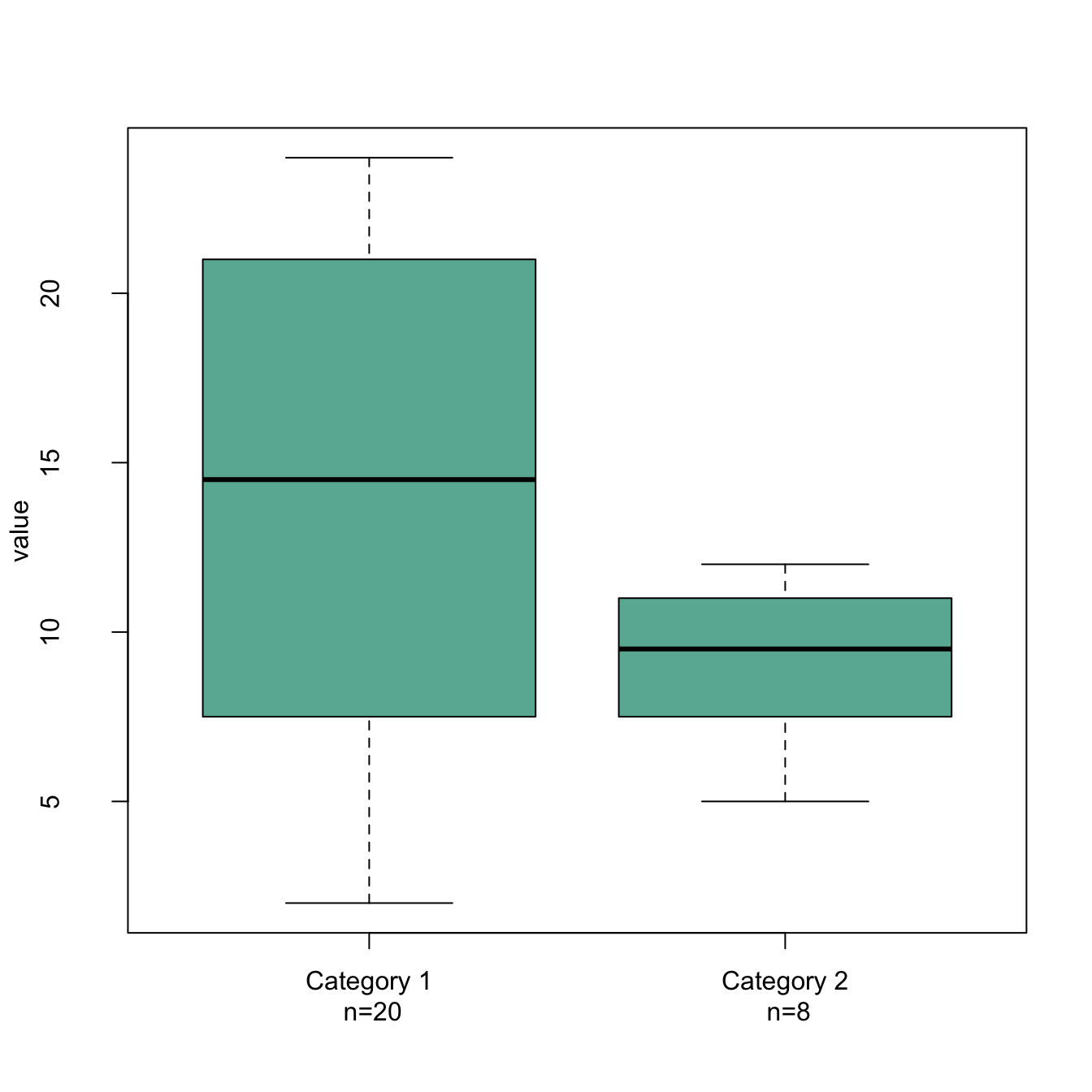# Basic R: X axis labels on several lines

It is a common practice to display the X axis label on several lines. Here is an example applied to a boxplot.

It can be handy to display X axis labels on several lines. For instance, to add the number of values present in each box of a boxplot.

How it works:

• Change the names of your categories using the `names()` function.
• Use `\n` to start new line
• Increase the distance between the labels and the X axis with the `mgp` argument of the `par()` function. It avoids overlap with the axis.

Note: `mgp` is a numeric vector of length 3, which sets the axis label locations relative to the edge of the inner plot window. Default value : c(3,1,0). First value : location the labels (xlab and ylab in plot). Second value : location of the tick-mark labels (what we want to lower). Third Value : position of the tick marks``````# Create 2 vectors
a <- sample(2:24, 20 , replace=T)
b <- sample(4:14, 8 , replace=T)

# Make a list of these 2 vectors
C <- list(a,b)

# Change the names of the elements of the list :
names(C) <- c(paste("Category 1\n n=" , length(a) , sep=""), paste("Category 2\n n=" , length(b) , sep=""))

# Change the mgp argument: avoid text overlaps axis
par(mgp=c(3,2,0))

# Final Boxplot
boxplot(C , col="#69b3a2" , ylab="value" )``````

Related chart types

## Contact

This document is a work by Yan Holtz. Any feedback is highly encouraged. You can fill an issue on Github, drop me a message on Twitter, or send an email pasting yan.holtz.data with gmail.com.